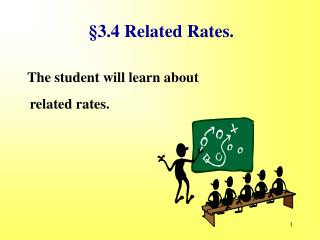DownloadDownload Presentation§3.4 Related Rates.

§3.4 Related Rates.

Télécharger la présentation§3.4 Related Rates.

- - - - - - - - - - - - - - - - - - - - - - - - - - - E N D - - - - - - - - - - - - - - - - - - - - - - - - - - -
Presentation Transcript

1. §3.4 Related Rates. The student will learn about related rates.

2. Introduction to Related Rates All of our relationships involve two variables. We have found the derivative with respect to one of those variables in order to find a rate of change in that variable. Related rate problems find the rate of change of both variables (implicitly) with respect to time and solve for one rate with respect to the other.

3. z dy/dt is given as 5 meters per second. y 300 Example 1 A weather balloon is rising vertically at the rate of 5 meters per second. An observer is standing on the ground 300 meters from the point where the balloon was released. At what rate is the distance between the observer and the balloon changing when the balloon is 400 meters high? Consider making a drawing. Note from the drawing that y and z are the variables. dz/dt is the unknown. What is an equation that relates y and z? z 2 = y 2 + 300 2

4. z y 300 Example 1 - continued A weather balloon is rising vertically at the rate of 5 meters per second. An observer is standing on the ground 300 meters from the point where the balloon was released. At what rate is the distance between the observer and the balloon changing when the balloon is 400 meters high? z 2 = y 2 + 300 2 Implicitly differentiate the equation. and solving for dz/dt yields,

5. z 2 = 400 2 + 300 2 z y 300 Example 1 - continued A weather balloon is rising vertically at the rate of 5 meters per second. An observer is standing on the ground 300 meters from the point where the balloon was released. At what rate is the distance between the observer and the balloon changing when the balloon is 400 meters high? When y = 400, z = 500. Why? And we know dy/dt = 5 meters/sec.

6. R Example 2 A rock is thrown into a still pond and causes a circular ripple. If the radius of the ripple is increasing at 2 feet per second, how fast is the area changing when the radius is 10 feet? [Use A = π R 2 ] Making a drawing. Note dr/dt = 2 and R = 10 are given. Find dA/dt. A = π R 2 Substitute what we know.

7. Solving Related Rate Problems Step 1. Identify the quantities that are changing with time. Express these rates as derivatives. Step 2. Identify all variables, including those that are given and those to be found. Step 3.Find an equation connecting variables. Sketch a figure if helpful. Step 4.Implicitly differentiate this equation. Step 5. Substitute into the new equation any given values for the variables and the derivatives. Step 6. Solve for the remaining derivative and interpret the answer as the unknown rate.

8. Example 3 The sides of a cube are increasing at 3 cm per second. How fast is the volume increasing when the edges are 5 cm? Making a drawing. Step 1. Identify the quantities that are changing with time. Express these rates as derivatives. s = 6 Step 2. Identify all variables, including those that are given and those to be found. s = side of the cube = 5 V = volume of cube = 5 3 = 125 8

9. Example 3 s = 5 V = 125 The sides of a cube are increasing at 3 cm per second. How fast is the volume increasing when the edges are 6 cm? Step 3.Find an equation connecting variables. V = s 3 Step 4.Implicitly differentiate this equation. s = 6 Step 5. Substitute into the new equation any given values for the variables and the derivatives. 9

10. Example 3 s = 5 V = 125 The sides of a cube are increasing at 3 cm per second. How fast is the volume increasing when the edges are 6 cm? Step 6. Solve for the remaining derivative and interpret the answer as the unknown rate. s = 6 Notice you do not need V in the problem. Did you get the units correct? Do you understand the 225 cm 3 / second? 10

11. Summary. • We learned that related rate problems occur in life and in business and they need calculus for their solutions.

12. ASSIGNMENT §3.5; Page 65; 1 - 9, odd.